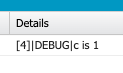# Found punctuation symbol or operator ‘%’ that isn’t valid in Apex% cannot be used in Apex. But one should use Math.mod() instead.
% is not a valid operator in Apex. So, use Math.mod() instead of % operator.
Sample Code:
Integer a = 10;
Integer b = 3;
Integer c = Math.mod( a, b );
System.debug( ‘c is ‘ + c );
Output: